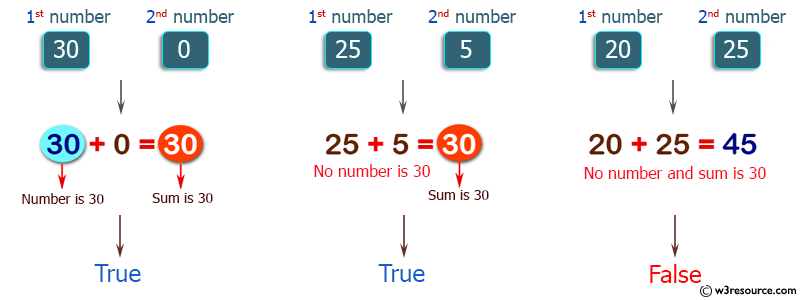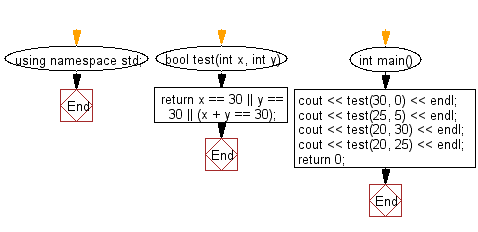﻿ C++ : If either of two integers is 30 or if their sum is 30# C++ Exercises: Check two given integers, and return true if one of them is 30 or if their sum is 30

## C++ Basic Algorithm: Exercise-3 with Solution

Write a C++ program to check two given integers, and return true if one of them is 30 or if their sum is 30.

Sample Solution:

C++ Code :

``````#include <iostream>
using namespace std;

bool test(int x, int y)
{
return x == 30 || y == 30 || (x + y == 30);
}

int main()
{
cout << test(30, 0) << endl;
cout << test(25, 5) << endl;
cout << test(20, 30) << endl;
cout << test(20, 25) << endl;
return 0;
}
``````

Sample Output:

```1
1
1
0
```

Pictorial Presentation:Flowchart:C++ Code Editor:

Contribute your code and comments through Disqus.

What is the difficulty level of this exercise?

﻿